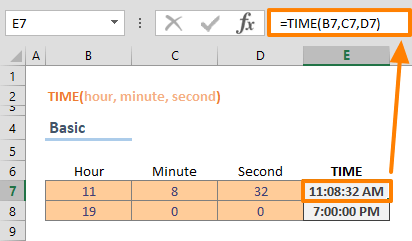The Excel TIME function is a Date & Time formula that calculates and returns the serial time value based on the specified hour, minute, and second values. In this guide, we’re going to show you how to use the Excel TIME function and also go over some tips and error handling methods.

## Supported versions

• All Excel versions

## Excel TIME Function Syntax

TIME(hour, minute, second)

## Arguments

 hour The numeric value for hours, from 0 (zero) to 32767 minute The numeric value for minutes second The numeric value for seconds

## Excel TIME Function Examples

### Basic use case

Each argument of the Excel TIME function is required to get the time value. In a typical use case, you need to enter the integer values for hour, minute, and second arguments. For example, to generate a time serial value for 11:08:32, you can use the following formula:

=TIME(11,8,32)In fact, Excel returns 0.464259259259259 value from the above formula. However, the value is displayed in a time format.

### Other use cases

Another common way of using the Excel TIME function is for finding the values between known limits for the arguments. While the hour can be between 0 and 23, the minute and the second can be anywhere between 0 and 59. These numbers are natural limits for a time value on the 24-hour-system. However, you can override these limits by using negative, or larger numbers.

When Excel detects a number larger than the natural limits, e.g. 75 for minute, it adds the difference to the next time argument, which would be hour in this case.

For example, the following formula returns the serial value for time 2:15:45

=TIME(1,75,45)

In the same manner, negative numbers are subtracted from the larger number when used for any of the arguments. For example, if you enter -85 into the second argument, Excel subtracts that value from the minute and hour, if necessary.

=TIME(4,30,-85)## Tips

• Excel stores date and time as numbers. Excel assumes that the date 1/1/1900 is “1″, and increases this number by 1 for each day. In a similar pattern, the time values can be between 0 and 0.999999999. For example; 6:00 AM is equal to 25. Because of this, the Excel TIME function returns a number that is displayed as a time value.
• You can use negative values, unless the return value would give a negative value.

## Issues

• The TIME function returns #NUM! error, if return value is negative.